## 7.1 - Introduction to graph theory: basic concepts

• Computer scientists create abstractions of real world problems for representing and manipulating with a computer.
• One example, is logic which is used to define a computer circuits.
• Scheduling final exams is another example:
• Has to take into account associations between courses, students and rooms.
• These associations (connections) between items are modelled by graphs.
• Graphs are discrete structures consisting of vertices (nodes) and edges connecting them.
• Graph theory is an area in discrete math which studies these type of discrete structure.
• What is a graph?
• Graphs are discrete structures consisting of vertices (nodes) and edges connecting them.
• Graph theory is an area that studies these structures.
• Origins of graph theory:
• First problem in graph theory is Seven Bridges of Konigsbert problem solved by Loenhard Euler in 1735.
• 2 islands are connected by 7 bridges.
• Can you walk across all 7 bridges only once.
• In a paper published in 1726, he showed that it is impossible.
• Applications of graphs
• Used in a variety of disciplines.
• Examples:
• Networks.
• Solving shortest path problems between cities.
• Assigning jobs to employees in an organisation.
• Distinguishing chemical compound structures.

## Lesson 7.103 - Definition of a graph

• Graph
• Discrete structures consisting of vertices and edges connecting them.
• Formal definition:
• $G$ is an ordered pair $G=(V, E)$.
• $V$ is a set of nodes, or vertices.
• $E$ is a set of edges, lines or connections.
• Vertex

• Basic element of a graph.
• Drawn as a node or a dot.
• Set of vertices of G is usually denoted by V(G) or V.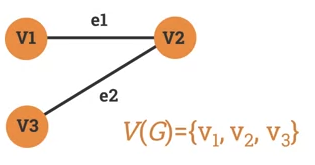• Edge

• A is a link between 2 vertices.
• Drawn as a line connecting two vertices.
• A set of edges in a graph $G$ is usually denoted by $E(G)$ or $E$.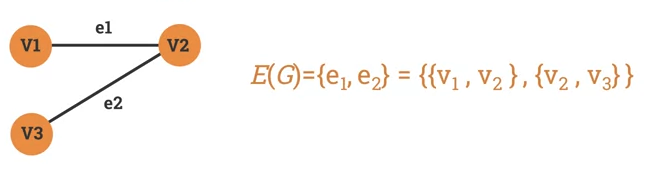• Two vertices are said to be adjacent if they are endpoints of the same edge.
• Two edges are said adjacent if they share the same vertex.
• If a vertex v is an endpoint of an edge e, then we say that e and v are incident.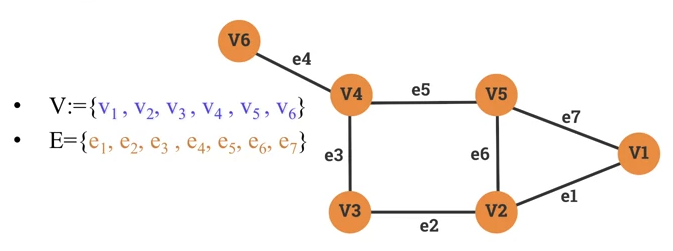• $v_1$, $v_2$ are endpoints of the edge $e_1$. We say that $v_1$ and $v_2$ are adjacent.

• The edges $e_1$ and $e_7$ share the same vertex $v_1$. We say that $e_1$ and $e_7$ are adjacent.
• The vertex $v_2$ is an endpoint of the edge $e_1$. We say that $e_1$ and $v_2$ are incident.
• Loops and parallel edges

• Consider this graph: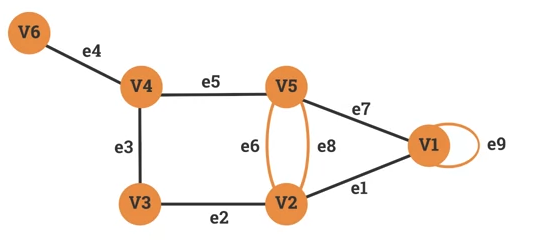• $v_2$ and $v_5$ are linked with 2 edged: (e_6 and e_8). e_6 and e_8 are considered Parallel Edges.

• $v_1$ is linked by $e_9$. We call the edge $e_9$ a Loop.
• Directed Graphs
• Aka digraph.
• Graph where edges have a direction.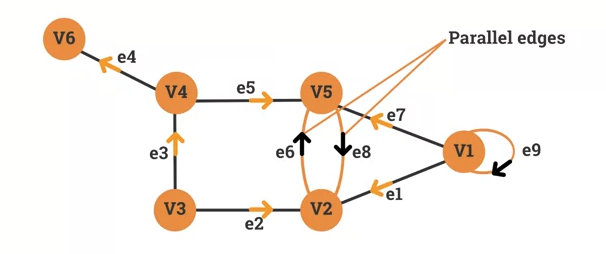• $e_1$ is a connection from $v_1$ to $v_2$ but not from $v_2$ to $v_1$

• $e_6$ is a connection from $v_2$ to $v_5$ whereas $e_8$ is a connection from $v_5$ to $v_2$.

## Lesson 7.105 Walks and paths in a graph

• Definition of a Graph Walk

• Sequences of vertices and edges of a graph.
• Vertices and edges can be repeated.
• A walk of length k in a graph is a succession of $k$ (not necessarily different) edges of form:
• $uv$, $vw$, $wx$, ..., $yz$
• Example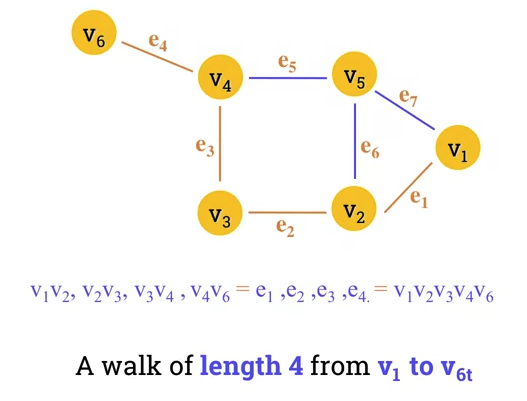• Example 2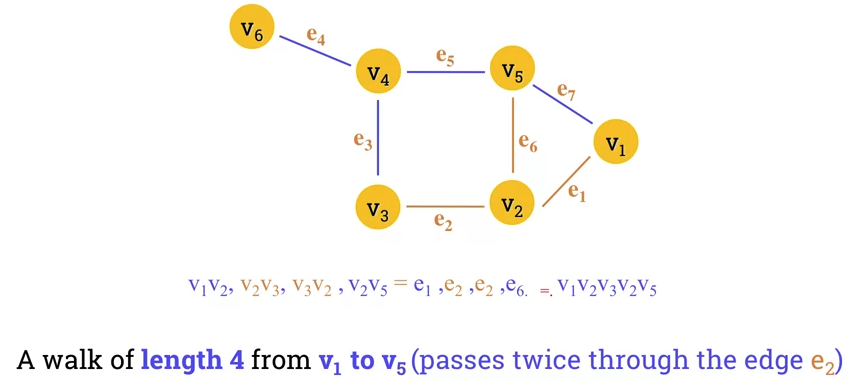• Graph Trail

• A trail is a walk where no edge is repeated.
• In a trail, vertices can be repeated but no edge is repeated.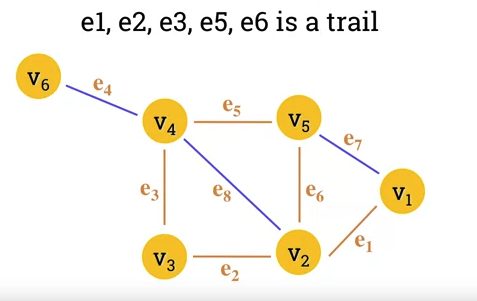• Graph Circuit

• A circuit is a closed trail.
• Circuits can have repeated vertices only.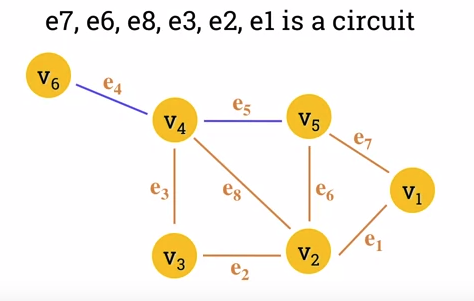• Graph Path

• A path is a trail in which neither vertices nor edges are repeated.
• Length of path is given in number of edges it contains.
• Graph Cycle

• Closed path consisting of edges and vertices where a vertex is reachable from itself.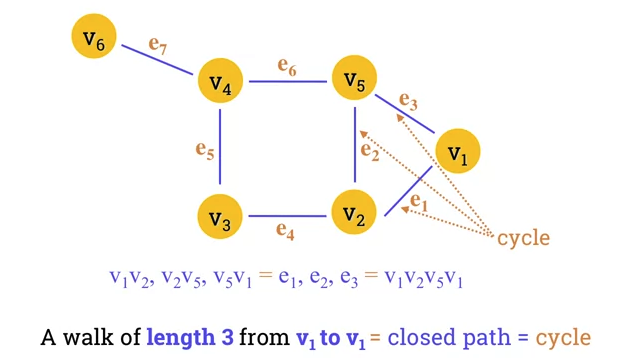• Seven Bridges of Koenigsberg

• Is there a walk that passes each of the 7 bridges once.
• No walk that uses each edge exactly once (even if we allow the walk to start and finish in diff places)
• Euler's Path

• A Eulerian path in a graph is a path that uses each edge precisely once.

• If the path exists, the graph is called traversable.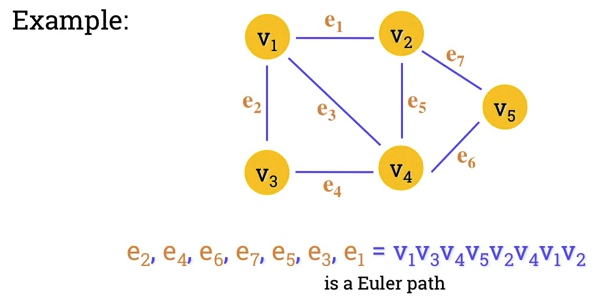• Hamiltonian path

• Hamiltonian path (aka traceable path) is a path that visits each vertex exactly once.
• A graph that contains a Hamiltonian path is called a traceable graph.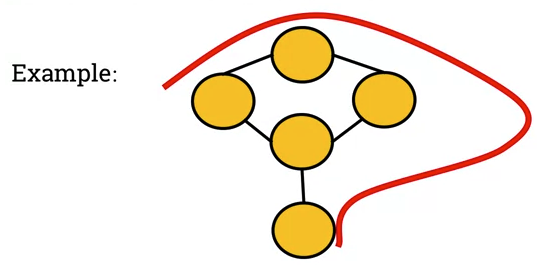• Hamiltonian cycle

• A Hamilton cycle is a cycle that visits each vertex exactly once (except for the starting vertex, which is visited once at the start and once again at end).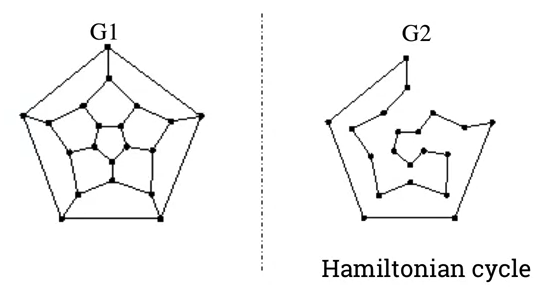• Connectivity

• An undirect graph is connected if
• You can get from any node to any other by following a sequence of edges OR
• any two nodes are connected by a path.
• Example of connected graph: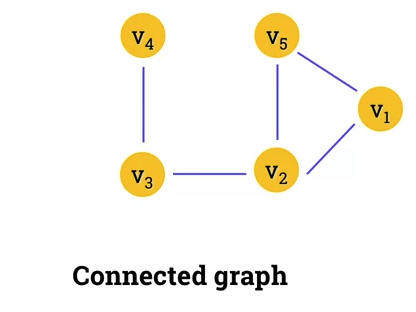• Unconnected graph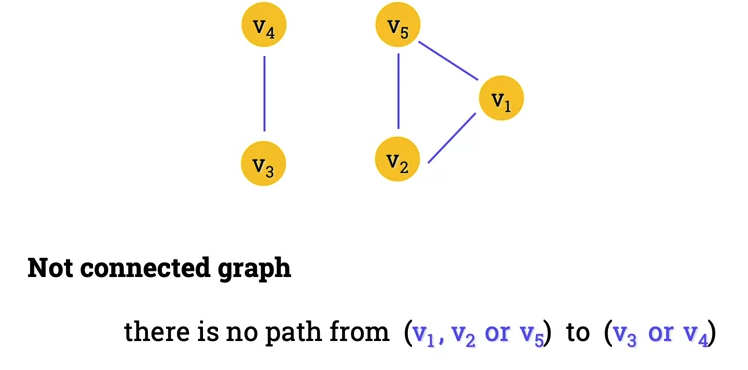• Strong connectivitiy

• A directed graph is strongly connected if there is a directed path from any node to any other node.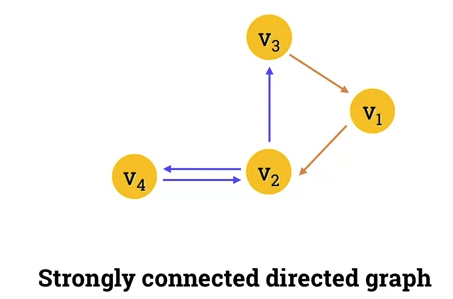• Example of a graph that's not strongly connected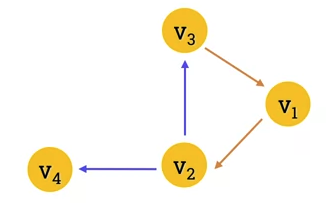• No direct path from v_4 to any of the other 3 vertices.
• Transitive Closure
• Given a digraph G, the transitive closure of G is the digraph G such that: G has the same verticies as G
• If G has a directed path from $u$ to $v$ ($u \ge v$), G* has a directed edge from $u$ to $v$.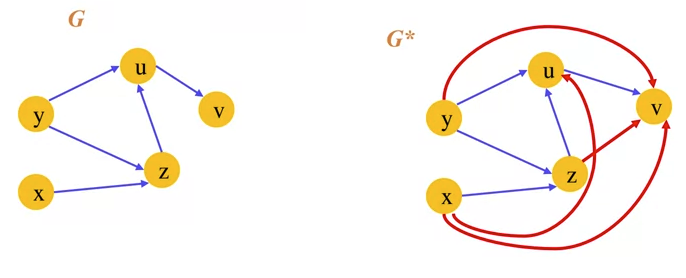• Transitive closure provides reachability information about a digraph.

## Lesson 7.107 - The degree sequence of a graph

• Degree of Vertices

• The number of edges incident on v.
• A loop contributes twice to the degree.
• An isolated vertex has a degree of 0.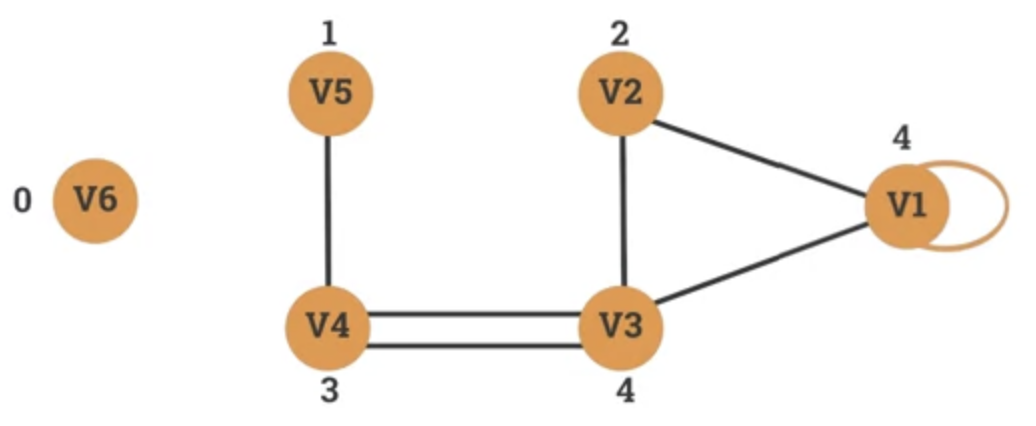• For directed graphs:

• In-deg (v): number of edges for which v is the terminal vertex.
• Out-deg (v): number of edges for which v is the initial vertex.
• deg(v) = Out-deg(v) + In-deg(v)
• A loop contributes twice to the degree, as it contributes 1 to both in-degree and out-degree.
• Degree Sequence of a Graph

• Given an undirected graph G, a degree sequence is a monotonic nonincreasing sequence of the vertex degress of all the vertices G.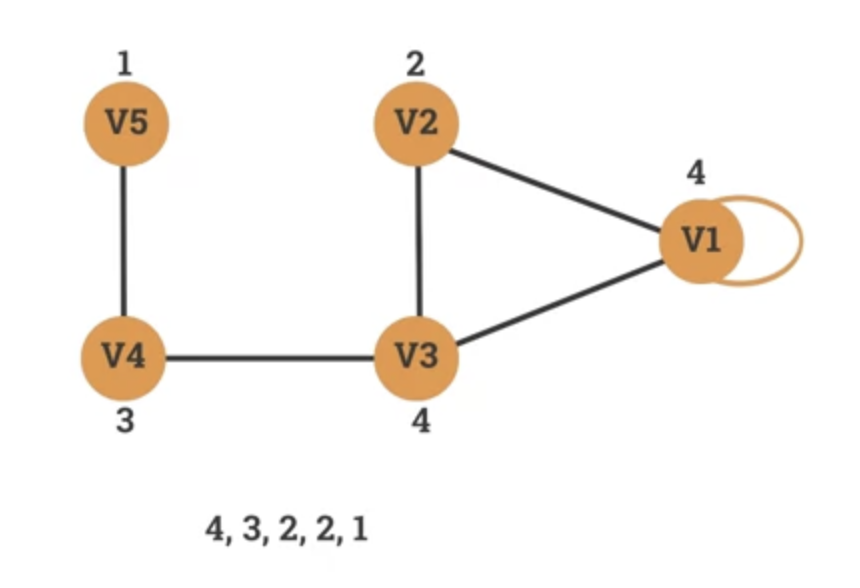• Properties of graph degree sequence:

• Degree sequence property 1
• The sum of degree sequence of a graph is always even.
• It is impossible to construct a graph where the sum of degree sequence is odd.
• Degree sequence property 2

• Given a graph G, the sum of the degree sequence of G is twice the number of edges in G.
• Number of edges(G) = (sum of degree sequences of G) / 2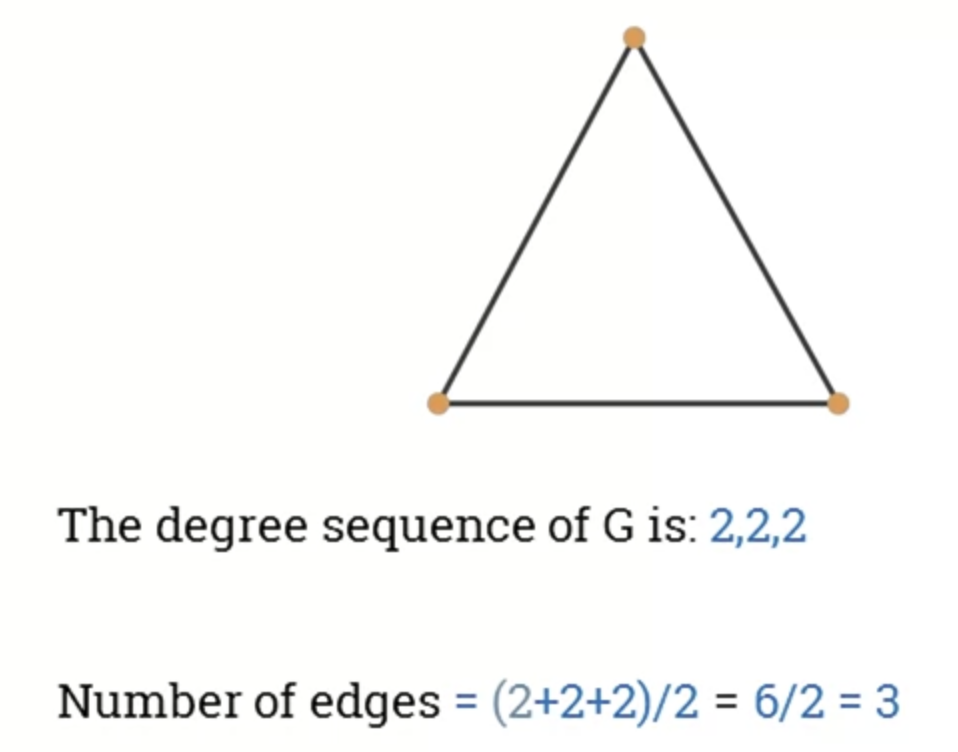• Exercise: which of the 2 degree sequences below is it possible to construct a graph with?

• 3, 2, 2, 1
• Sum of sequence = 3 + 2 + 2 =1 = 8
• Can build a graph with this.
• Number of edges = 8/2 = 4
• 3, 3, 2, 1
• Sum of sequence = 3 + 3 + 2 + 1 = 9
• Can't build a graph with this.

## 7.109 - Special graphs: simple, r-regular and complete graphs

• Simple Graph

• A graph without loops and parallel edges.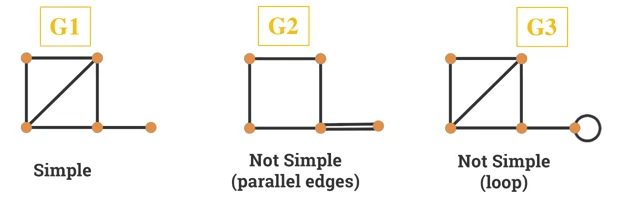• Given a simple graph G with n vertices, then the degree of each vertex of G is at most equal to n-1.
• Proof
• Let $v$ be a vertex of G such that deg(v) > n-1
• However, we have only n-1 other vertices for v to be connected to.
• Hence, the other connections can only be a result of parallel edges or loops
• Exercise
• Can we draw a simple graph with the following degree sequences?
• 4, 2, 2, 2
• Sum(deg sequence) is even so we can construct a graph
• However, no other vertex has degree of 4. There are only 3 other vertices to be connected to, so it has to contain a loop or parallel edges.
• 4, 3, 3, 2, 2
• Yes, it can be done.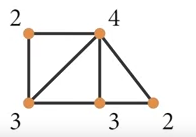• A graph is regular if all local degrees are the same number.
• A graph G where all vertices the same degree, $r$, is called a r-regular graph.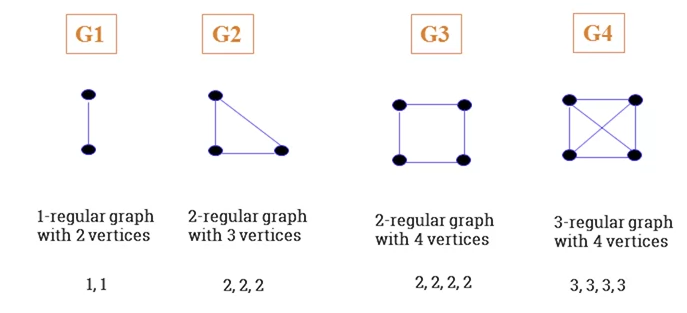• Given a r-regular G with n vertices, then the following is true:

• Degree sequence of $G = r, r, r, ..., r (\text{n times})$
• Sum of degree sequence of $G = r \ x \ n$
• Number of edges in $G = \frac{r \ x \ n}{2}$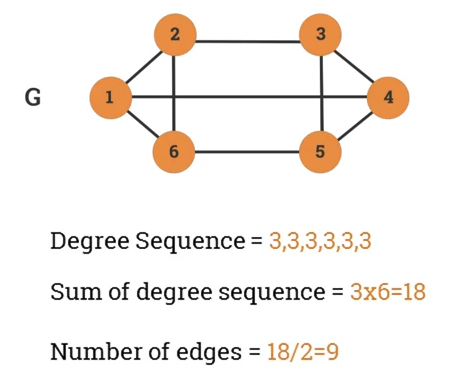• Special regular graphs: cycles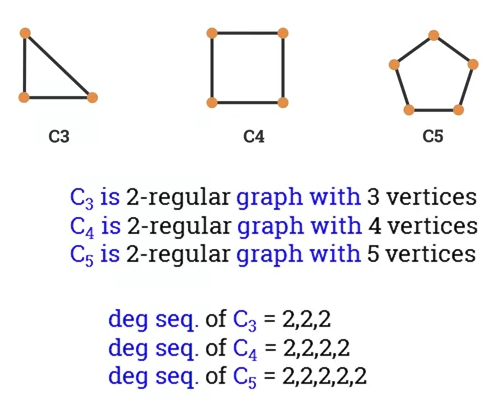• Exercise
• Can we construct a 3-regular graph with 4 vertices?
• Can we construct a 4-regular graph with 5 vertices?
• 3x5 = 15
• Sum is odd, so cannot great a regular graph.
• Complete Graph

• A Simple Graph where every pair of vertices are adjacent (linked with an edge).
• A vertex on its own is a complete graph.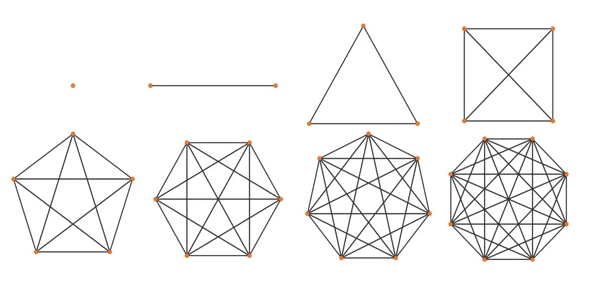• A complete graph with n vertices, k_n, has these properties:
• Every vertex has a degree $(n-1)$
• Sum of degree sequence $n(n-1)$
• Number of edges $n(n-1)/2$